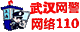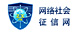## 2019年成人高考高起点文数考试章节难点解析（六）

●难点磁场

(★★★★★)设m是实数，记M={m|m>1},f(x)=log3(x2-4mx+4m2+m+ ).

(1)证明：当m∈M时，f(x)对所有实数都有意义;反之，若f(x)对所有实数x都有意义，则m∈M.

(2)当m∈M时，求函数f(x)的最小值.

(3)求证：对每个m∈M,函数f(x)的最小值都不小于1.

●案例探究

[例1]设计一幅宣传画，要求画面面积为4840 cm2,画面的宽与高的比为λ(λ<1),画面的上、下各留8 cm的空白，左右各留5 cm空白，怎样确定画面的高与宽尺寸，才能使宣传画所用纸张面积最小?如果要求λ∈[ ]，那么λ为何值时，能使宣传画所用纸张面积最小?

∴S(λ1)-S(λ2)<0,∴S(λ)在区间[ ]内单调递增.

[例2]已知函数f(x)= ,x∈[1,+∞ (1)当a= 时，求函数f(x)的最小值.

(2)若对任意x∈[1,+∞ ,f(x)>0恒成立，试求实数a的取值范围.

(1)解：当a= 时，f(x)=x+ +2

∵f(x)在区间[1，+∞ 上为增函数，

∴f(x)在区间[1，+∞ 上的最小值为f(1)= .

(2)解法一：在区间[1，+∞ 上，f(x)= >0恒成立 x2+2x+a>0恒成立.

∴当x=1时，ymin=3+a,当且仅当ymin=3+a>0时，函数f(x)>0恒成立，故a>-3.

●锦囊妙计

(1)求函数的值域

(2)函数的综合性题目

(3)运用函数的值域解决实际问题QQ群号：387225515